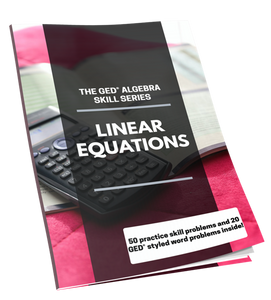GED Teacher

# The GED Algebra Skill Series: Linear Equations

Regular price \$ 15.00

55% of the GED® Math Test questions are algebra skill and word problem questions. There are three minimum algebra skills that are required for student success on the math test. These skills are:

1. Positive and Negative Numbers
2. Solving for X
3. Linear Equations

The GED® Algebra Skill Series is a three part workbook series—each workbook covers one of the three individual minimum algebra skills.

The GED® Algebra Skill Series: Linear Equations includes:

A winning strategy to pass the math test

A GED® Math Test overview

Plotting and Identifying Points on the Graph

Finding the Slope

Graphing the Line of an Equation

Writing an the Equation of a Line

Word problem exercises focused on processing development

A free video channel demonstrating 10 workbook problems

This workbook ships to you within 1-2 business days via USPS.